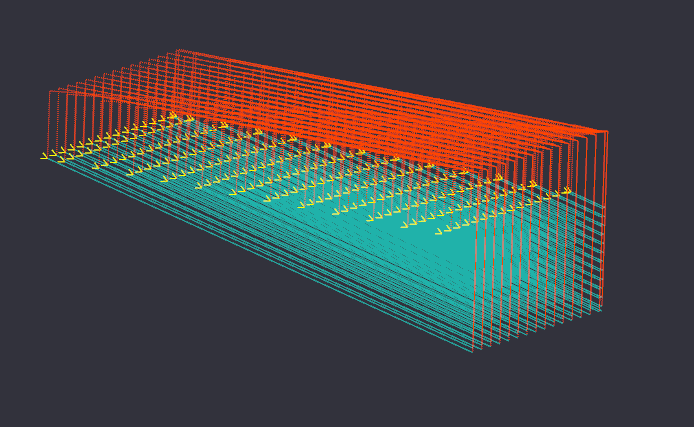# 30 September 2011 Sloped Lines VBScript

This script was created in response to query on how to generate a toolpath for a slot that slopes along the Z axis.

The script will generate a sequence of polylines that can be used as the source for an engraving operation.

The script was extended so that it can also produce a series of parallel sloped lines to machine a sloped surface.

The following parameters are used to control the script:

x1, y1 : The coordinates of the first control point.
x2, y2 : The coordinates of the second control point.
Target Depth : The deepest Z coordinate of the slot or surface.
Depth Increment : The maximum depth per cut.
Width : The width of the surface to cut. Use 0 to machine a slot. The tool diameter is not taken into account.
Stepover : The distance between each parallel cut for a surface.

Once the polylines are created, select them all, then insert an Engraving machine operation.

The engraving operation should have Target Depth set to 0 and Optimisation Mode set to None, so that the machining operation will follow the source polylines exactly, in the order they were created.VBScript:

```sub main

' First line point
dim x1 as double = 40
dim y1 as double = -20

' Second line point
dim x2 as double = 60
dim y2 as double = -30

dim TargetDepth as double = -5
dim DepthIncrement as double = 0.4

dim Width as double = 10
dim Stepover as double = 0.7

dim z as double = 0

dim v as Vector2F = new Vector2F(x1-x2,y1-y2)
dim vunit = v.Unit()
dim vnorm = vunit.Normal()

dim px1, px2, py1, py2 as double

do
z = z - DepthIncrement
if z < TargetDepth then z = TargetDepth

dim w as double = 0

dim l as double = v.Length * Math.Abs(z) / Math.Abs(TargetDepth)

do
dim poly as polyline =new Polyline()

px1 = (x2 + vunit.X * l) + vnorm.X * w
px2 = x2 + vnorm.X * w

py1 = (y2 + vunit.Y * l) + vnorm.Y * w
py2 = y2 + vnorm.Y * w

if w = Width then exit do
w = w + Stepover
if w > Width then w = width

loop while w <= Width

loop while z > TargetDepth

end sub```

<< 1 >>
#  Steve Wolodkin 23/08/2016 22:07:11
I've been evaluating CamBam and I'm going to purchase a license. It would be advantageous to me to have Ramping as a supported milling strategy. What would it take to alter the Sloped Lines VB Script to accomplish this?

Thanks.